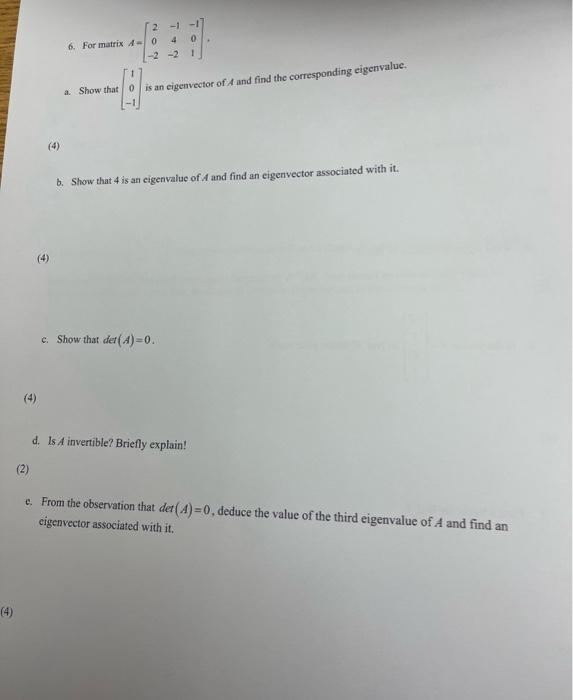# (Solved): 6. For matrix A=202142101. a. Show that 101 ...6. For matrix . a. Show that is an eigenvector of and find the corresponding eigenvalue. (4) b. Show that 4 is an eigenvalue of and find an eigenvector associated with it. (4) c. Show that . (4) d. Is invertible? Briefly explain! e. From the observation that , deduce the value of the third eigenvalue of and find an eigenvector associated with it.

We have an Answer from Expert

(a)Characteristic equation

(2-  ){(4-  (1-

Thus, the EIGEN VALUE OF MATRIX A are 0,3,4
when   the corresponding eigen vector is given by

-x-y-z=0 .......(1)
0.x+y+0.z=0 ..........(2)
-2x-2y-2z=0 ......(3)
from eqn.(2)
y=0
put y=0 in eqn.(3)
then we get -x-z=0
-x=z .....(4)
therefore put x=1 in eqn.(4) we get z=-1
then the eigen vector=  hence proved.

We have an Answer from Expert